SEARCH HOMEMath Central Quandaries & QueriesQuestion from Jonathan: I'm having a hard time making a design pattern for a cone sleeve, the thing is the cone sleeve is 22 degrees, how can i know the angle of this when it is flat on paper, based on my calculations, it should be around 66 - 69, but i want it to be exact can anybody help?Jonathan,

I have drawn what I see as your cone sleeve. Do I have the angle correct?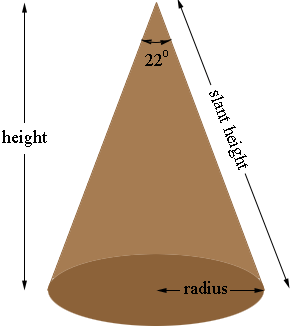I need some additional information. Can you tell me the radius and either the height or the slant height?

Penny

Jon wrote back

The 22 degrees is correct however the slant height, radius and height will vary on the length of the sleeve i will make, but the 22 degrees is constant what I like to know is if i cut this conical sleeve, and place it flat on a table, what will be the angle, the degrees will change also sometimes I design a 25 degrees or 18 degrees cone sleeve, all I want to know what is the angle if i cut and place them flat on a a surface.

- Jon

I redrew my diagram and labeled it. I changed the angle measurement from 22o to to so that what I do applied to other sleeves as well.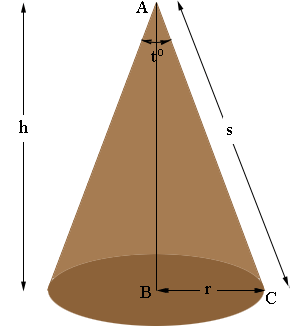The circumference of the circle at the base of the cone is 2 π r units.

When you slice the cone and roll it out flat you get a sector of a circle of radius s units and a central angle that measures wo.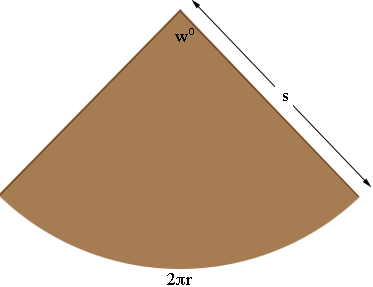The circle of radius s has a circumference of 2 π s units and the arc of length 2 π r is a portion of that circumference just as wo is a portion of the entire central angle of the circle of 3600. In fact by the symmetry of the circle these proportions are the same. That is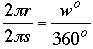or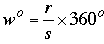This give you the angle measurement you require from r and s. If you can't measure the radius of the base r or the slant height s directly you can calculate them using the trigonometric relations relating r, s and h.

r = h × tan(t/2)
h = s × cos(t/2)

r = s × sin(t/2).

I hope this helps,
PennyMath Central is supported by the University of Regina and The Pacific Institute for the Mathematical Sciences.